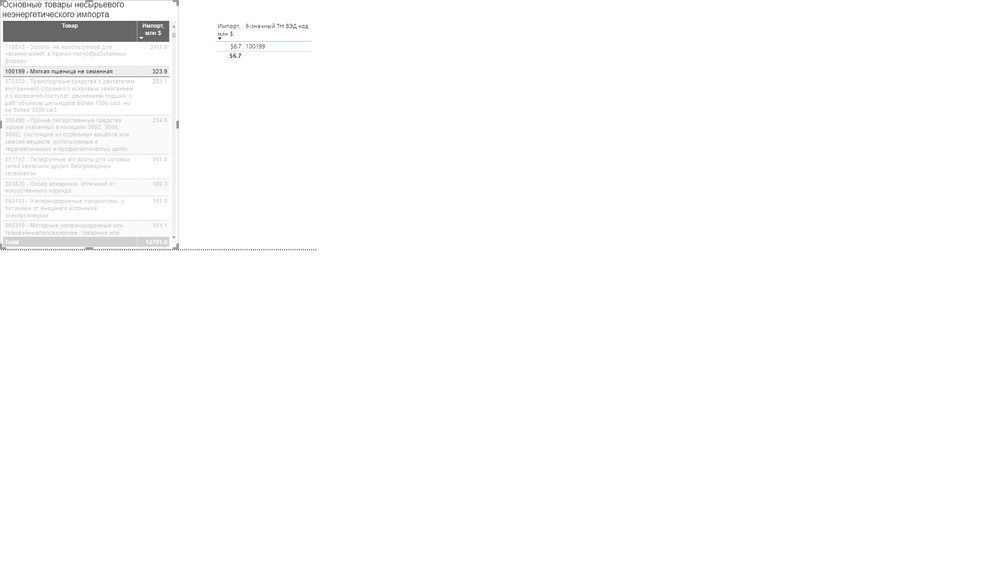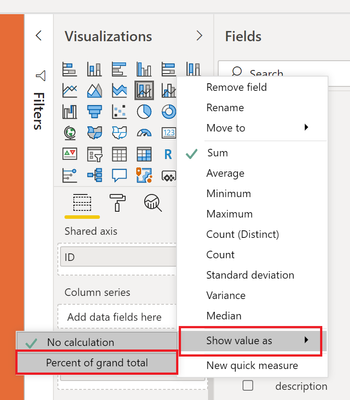cancel
Showing results for
Did you mean:Anonymous
Not applicable

## How to find share of the specific country for a specific products from overall value?

Dear all,

I am analyzing the import/export trade of the countries and I would like to get a share of the country partner for a specific product.

For example, in the figure below for the product 100199, the total was 323.9 \$ in 2019, and 56.7 \$ came from my country. In addition to this value, I would like to get a percentage. In this example, the share is 17.5%. So I want to see 56.7\$ and 17.5% together in one visual. How can I get this percentage value? So my inputs are: Product, Import Value, Reporter Country(imported country), and Partner country(is equal to my country).1 ACCEPTED SOLUTIONCommunity Support

Hi @Anonymous ,

You may create measure like DAX below.

``````Percentage =
DIVIDE (
CALCULATE (
SUM ( Table1[Import Value] ),
FILTER (
ALLSELECTED ( Table1 ),
Table1[Reporter Country] = MAX ( Table1[Reporter Country] )
&& Table1[Product] = MAX ( Table1[Product] )
)
),
CALCULATE (
SUM ( Table1[Import Value] ),
FILTER (
ALLSELECTED ( Table1 ),
Table1[Reporter Country] = MAX ( Table1[Reporter Country] )
)
)
)
``````

Best Regards,

Amy

Community Support Team _ Amy

If this post helps, then please consider Accept it as the solution to help the other members find it more quickly.

6 REPLIES 6Super User

@Anonymous - Add your measure/column to your table/matrix again. In the Visualizations area, click the drop down arrow for the field and choose Show As | Percent of Grand TotalBecome an expert!: Enterprise DNA
External Tools: MSHGQM
Latest book!:
Mastering Power BI 2nd EditionDAX is easy, CALCULATE makes DAX hard...Anonymous
Not applicable

Thx for reply, but this method is showing total as 100%. I am sure that there should be a measure to calculate @Greg_DecklerSuper User

@Anonymous - Probably need to better understand your source data. Please post text sample. Percentages are covered in recipe 7 of chapter 4 of DAX Cookbook here: https://github.com/gdeckler/DAXCookbook

Become an expert!: Enterprise DNA
External Tools: MSHGQM
Latest book!:
Mastering Power BI 2nd EditionDAX is easy, CALCULATE makes DAX hard...Super User

@Anonymous ,

You can have measure like

divide(sum(Table[value]),calculate(sum(Table[value]),allselected(Table)))

or

divide(sum(Table[value]),calculate(sum(Table[value]),all(Table)))Anonymous
Not applicable

Thx for your answer, unfortunately, these measures did not work. I want to see the import  share of my country for a specific product as I mentioned before.  @amitchandakCommunity Support

Hi @Anonymous ,

You may create measure like DAX below.

``````Percentage =
DIVIDE (
CALCULATE (
SUM ( Table1[Import Value] ),
FILTER (
ALLSELECTED ( Table1 ),
Table1[Reporter Country] = MAX ( Table1[Reporter Country] )
&& Table1[Product] = MAX ( Table1[Product] )
)
),
CALCULATE (
SUM ( Table1[Import Value] ),
FILTER (
ALLSELECTED ( Table1 ),
Table1[Reporter Country] = MAX ( Table1[Reporter Country] )
)
)
)
``````

Best Regards,

Amy

Community Support Team _ Amy

If this post helps, then please consider Accept it as the solution to help the other members find it more quickly.